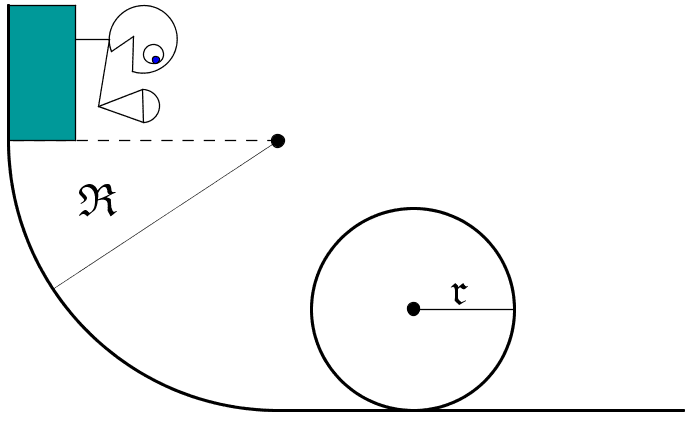# A boy, a cart, a loop , an icecream-cone

Was sitting in class thinking about this problem, did some rough sketches of a solution but never really managed to solve it.

![Ice cream cone and a loop-de-loop]Question:
Assume a boy starts at the top of a circle with radius $R$ as described in the picture. It is a snowy day and the path can be considered frictionless. The boy enters a loop with radius $r$ at the bottom of the hill. At the top of the loop the boy loses his icecream cone in such a way that it starts falling. The initial velocity of the ice-cream is $0$ m/s straight down.

The problem is to find $R$ expressed by $r$, such that the boy reaches the ice-cream just as he reaches the bottom of the loop​

The problem boiled down to finding out how much time the boy uses getting from the top of the loop to the bottom. Any help, solutions or inputs would be great.

--------------------------------------

My attempt, I know that this is most likely 90% wrong

By using conservation of mechanical energy. The speed at the bottom of the hill equals

$$v_b^2 = Rmg$$

And the velocity at the top of the loop equals

$$v_t^2 = 2g\left( R - 2r \right)$$

Vi know that the aceleration is constant and equals $g$ (Here is where I think I make my mistake, forgot to account for the angular velocity)

$$s = \dfrac{v_1 - v_0}{2} t$$

We use this equation to find out how long it takes the boy to get from the top, to the bottom of the loop.

$$t \, = \, \dfrac{2s}{v_1 - v_0} \, = \, \dfrac{2\left( \dfrac{2\pi r}{2}\right)}{\sqrt{2gR} - \sqrt{2g(R - 2r)}}$$

Now we figure out how long it takes the icream to fall the distance of the diameter or $2r$ .

$$s = v_0 + \dfrac{1}{2}gt^2$$
$$t = \sqrt{\dfrac{2s}{g}} \, = \, \sqrt{\dfrac{4r}{g}} \, = \, 2 \sqrt{\dfrac{r}{g}}$$

By setting these two equations equal each other, and solving for $R$ , we obtain that

$$R = \dfrac{16+8 \pi^2+\pi^4) r}{8 \pi^2} \cdot r \approx 2.43 r$$

------------------------------------------------------

Any comments, suggestions, hints soloutions would be much obliged =)